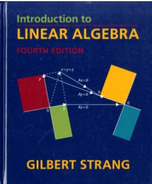Introduction To Linear Algebra - 4 Edition - Chapter 4.1 - Problem 1
Register Now

Join StudySoup

Get Full Access to Introduction To Linear Algebra - 4 Edition - Chapter 4.1 - Problem 19780980232714

# Get solution: Questions 1-12 grow out of Figures 4.2 and 4.3 with four subspaces

Introduction to Linear Algebra | 4th Edition

Problem 1

Questions 1-12 grow out of Figures 4.2 and 4.3 with four subspaces.

Accepted Solution
Step-by-Step Solution:
Step 1 of 3

L21 - 8 ex. The demand function for a certain product is given by the equation x p+24p =2 0,hedmand x is measured in hundreds of units. At what rate is the demand for the product changing when the current weekly demand is 400 units and the price is decreasing at the rate of 25 cents weekly

###### Chapter 4.1, Problem 1 is Solved

Step 2 of 3

Step 3 of 3

Unlock Textbook Solution